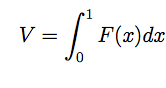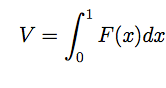# Volume of a rectangle through a sphere

• Locoism
In summary, -A square hole with sides of length 2 is cut symmetrically through the center of a sphere of radius 2.-The volume removed is given bywhere

## Homework Statement

Suppose that a square hole with sides of length 2 is cut symmetrically through
the center of a sphere of radius 2. Show that the volume removed is given bywhereI'm not sure how to approach this, but I figure you can express the sphere in terms of f(x,y,z) = $x^2+y^2+z^2=4$
then I express z in terms of x and y
$\sqrt{4-y^2-x^2}$

But now I'm guessing I need to set up a double integral. Looking at bounds, -2<x<2 and -1<y<1,

$\int_{-2}^{2}\int_{-1}^{1}y\sqrt{4-x^2-y^2}dydx$

Is this the right way to go?
How then would I show it is given by the above integral?

You are thinking about the hole going parallel to the z axis, right? Did you mean the integrand to be 2*sqrt(4-x^2-y^2)? And why should the x limits be different from the y limits if you want to cut out a square hole? The original question is a little confusing. You could just put F(x)=V. But I suspect they just want you do the dy integration and then use symmetry.

Locoism said:

## Homework Statement

Suppose that a square hole with sides of length 2 is cut symmetrically through
the center of a sphere of radius 2.
When you say "sides of length 2" do you mean the length of the hole through sphere or do you mean the lengtth of the edges where the hole comes out of the sphere?

In either case, I would calculate, first, the volume of the rectangular solid making up the hole. You don't need Calculus for that. Then I would calculate the volume of the two "caps" that are cut from the sphere. That will require a double integral.

Show that the volume removed is given bywhereI'm not sure how to approach this, but I figure you can express the sphere in terms of f(x,y,z) = $x^2+y^2+z^2=4$
then I express z in terms of x and y
$\sqrt{4-y^2-x^2}$

But now I'm guessing I need to set up a double integral. Looking at bounds, -2<x<2 and -1<y<1,

$\int_{-2}^{2}\int_{-1}^{1}y\sqrt{4-x^2-y^2}dydx$

Is this the right way to go?
How then would I show it is given by the above integral?
If the hole is of length 2, then the edges

Locoism said:
How then would I show it is given by the above integral?

Show, yeah, that's right. Now you livin' in the big house:

$$\text{myblackarea(x)}=\int_{-1}^{1} 2\sqrt{4-x^2-y^2}dy$$

$$\text{myvolume}=\int_{0}^{1} \text{2 myblackarea(x)}dx$$

And if you take the time to learn how to do this, you'll never have a problem with these integrals ever again. My point is, the act of creating the plot, cultivates an intutitive understanding of the underlying mathematics. So tell your teacher I said ask everyone in class to draw this picture even if it takes them 6 hours to figure out how.

Last edited: